Next: The approximating posterior distribution Up: Combining the two models Previous: Combining the two models   Contents

## The structure of the model

The probabilistic interpretation of the dynamics of the sources in the standard NSSM is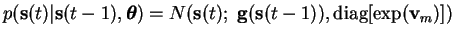(5.49)

where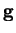is the nonlinear dynamical mapping andis the covariance matrix of the zero mean innovation process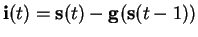. The typical linear approach, applied to the nonlinear case, would use a differentandfor all the different states of the HMM.

The simplified model uses only one dynamical mappingbut has an own covariance matrix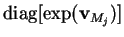for each HMM state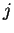. In addition to this, the innovation process is not assumed to be zero-mean but it has a mean depending on the HMM state. Mathematically this means that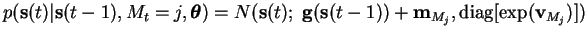(5.50)

where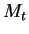is the HMM state,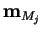andare, respectively, the mean and the covariance matrix of the innovation process for that state. The prior model of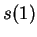remains unchanged.

Equation (5.50) summarises the differences between the switching NSSM and its components, as they were presented in Sections 5.1 and 5.2. The HMM output'' distribution is the one defined in Equation (5.50), not the data likelihood as in the stand-alone'' model. Similarly the model of the continuous hidden states in NSSM is slightly different from the one specified in Equation (5.25).Next: The approximating posterior distribution Up: Combining the two models Previous: Combining the two models   Contents
Antti Honkela 2001-05-30